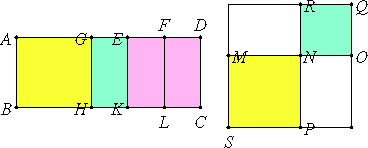# Proposition 56

If an area is contained by a rational straight line and the third binomial, then the side of the area is the irrational straight line called a second bimedial.

Let the area ABCD be contained by the rational straight line AB and the third binomial AD divided into its terms at E, of which terms AE is the greater.

I say that the side of the area AC is the irrational straight line called a second bimedial.

Make the same construction as before.X.Def.II.3

Now, since AD is a third binomial straight line, therefore AE and ED are rational straight lines commensurable in square only, the square on AE is greater than the square on ED by the square on a straight line commensurable with AE, and neither of the terms AE and ED is commensurable in length with AB.

Then, in manner similar to the foregoing, we shall prove that MO is the side of the area AC, and MN and NO are medial straight lines commensurable in square only, so that MO is bimedial.

It is next to be proved that it is also a second bimedial straight line.

X.13

Since DE is incommensurable in length with AB, that is, with EK, and DE is commensurable with EF, therefore EF is incommensurable in length with EK.

X.21

And they are rational, therefore FE and EK are rational straight lines commensurable in square only. Therefore EL, that is, MR, is medial.

X.38

And it is contained by MN and NO, therefore the rectangle MN by NO is medial. Therefore MO is a second bimedial straight line.

Therefore, if an area is contained by a rational straight line and the third binomial, then the side of the area is the irrational straight line called a second bimedial.

Q.E.D.

## Guide

This proposition is used in X.72.